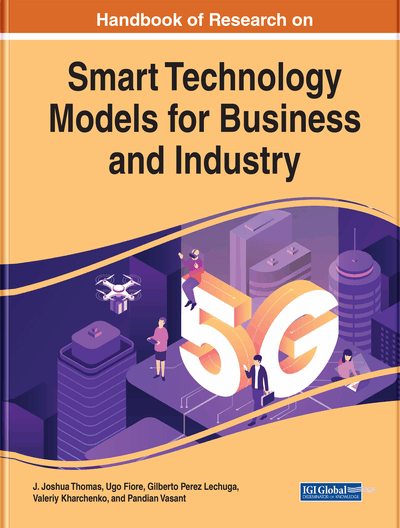# Application of Machine Learning Algorithms in Stock Market Prediction: A Comparative Analysis

Sumit Kumar, Sanlap Acharya
DOI: 10.4018/978-1-7998-3645-2.ch007
OnDemand:
(Individual Chapters)
Available
\$37.50
No Current Special Offers

## Abstract

The prediction of stock prices has always been a very challenging problem for investors. Using machine learning techniques to predict stock prices is also one of the favourite topics for academics working in this domain. This chapter discusses five supervised learning techniques and two unsupervised learning techniques to solve the problem of stock price prediction and has compared the performances of all the algorithms. Among the supervised learning techniques, Long Short-Term Memory (LSTM) algorithm performed better than the others whereas, among the unsupervised learning techniques, Restricted Boltzmann Machine (RBM) performed better. RBM is found to be performing even better than LSTM.
Chapter Preview
Top

## Introduction

In the stock market arena, markets show all the stock information that is currently available. This makes the task of stock price prediction almost impossible for investors, as the Efficient Market Hypothesis (EMH) would. Furthermore, the Random Walk Hypothesis (RWH) states that stock prices change in a stochastic manner. Over a long period of time, x has been used by the stock experts time-series prediction models to forecast stock prices. However, the reliability of all the hypotheses have been in question. There are some studies that have been successful in predicting stock prices to a certain extent. For example, Brock et al. (1992) predicted stock prices through moving averages and trading-range breaks. Both of them were technical trading rules, which means that x can use technical strategies to predict stock prices. More recent studies that use machine learning methods, such as artificial neural network, genetic algorithm, or support vector machines, argue that the market is inefficient at different levels in a wide range of stock markets, and therefore, it is feasible to predict the stock prices.

The authors’ objective is to predict the behavior of a financial time-series based on the present behavior with the help of various variables. Generally, x can classify such forecasting on the basis of explanatory variables into two categories: fundamental analysis and technical analysis. Sometimes, forecasters use techniques from both of these categories simultaneously, whereas there are cases in which x has used statistical models variables that fall under both categories. Along with statistical techniques, the authors observe the emergence of machine learning techniques to solve this problem. Sometimes, x uses a machine learning technique purely, and sometimes, x combines it with another statistical technique. One of the most popular machine learning techniques that has been widely used to predict financial time-series behavior is artificial neural network (ANN).

When x uses ANN for such predictions, selection of input or independent variables is as important an aspect as the structure of ANN. Two identical ANN models can show different outputs if their input variables are different. In this book chapter, the authors focus on the prediction accuracy and error of the models. In other words, a model having more accuracy and less error is more likely to be called an efficient model. However, an accurate model or a model that has fewer errors in the form of mean average deviation (MAD), root mean square error (RMSE), mean average error (MAE), mean square error (MSE) does not guarantee a profit in stock market (Leung et al., 2000). However, it may seem more likely that a model that has more accuracy and less error will be helpful for investors. Therefore, in this book chapter, the authors will be comparing two machine learning models that will predict future stock price.

This book chapter henceforth includes a brief literature review on prediction of stock prices using machine learning algorithms. The authors then briefly describe the various machine learning techniques. Next, the authors explain the dataset and discuss implementations of all the machine learning techniques. Then, the authors present the results, the discussion, the practical implications, and finally, the future scope in this domain.

## Complete Chapter List

Search this Book:
Reset